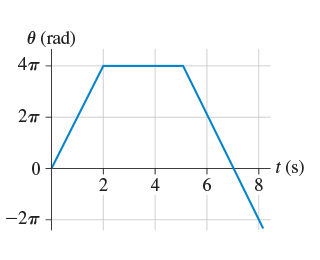# Problem: (Figure 1) shows the angular-position-versus-time graph for a particle moving in a circle.1.) What is the particle's angular velocity at t = 1 s? Express your answer using three significant figures.ω = rad/s2.) What is the particle's angular velocity at t = 4 s? Express your answer using three significant figures.ω = rad/s3.) What is the particle's angular velocity at t = 7 s? Express your answer using three significant figures.ω = rad/s

###### FREE Expert Solution

Angular velocity:

$\overline{){\mathbf{\omega }}{\mathbf{=}}\frac{\mathbf{∆}\mathbf{\theta }}{\mathbf{∆}\mathbf{t}}}$

The slope of an angular position versus time graph is the angular velocity.

1.

85% (355 ratings)###### Problem Details

(Figure 1) shows the angular-position-versus-time graph for a particle moving in a circle.1.) What is the particle's angular velocity at t = 1 s? Express your answer using three significant figures.

2.) What is the particle's angular velocity at t = 4 s? Express your answer using three significant figures.

3.) What is the particle's angular velocity at t = 7 s? Express your answer using three significant figures.